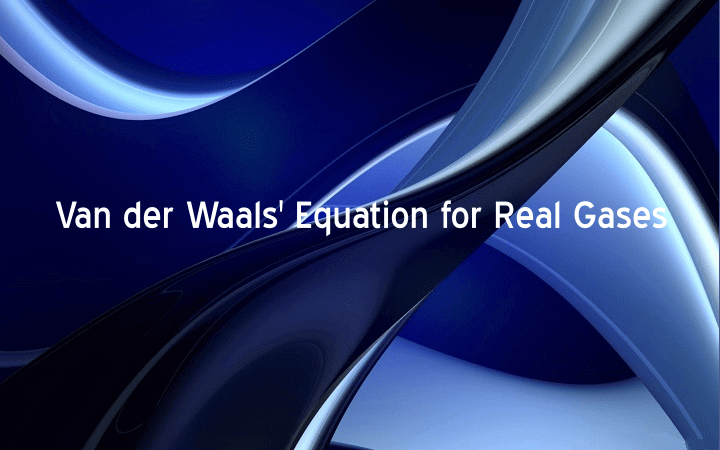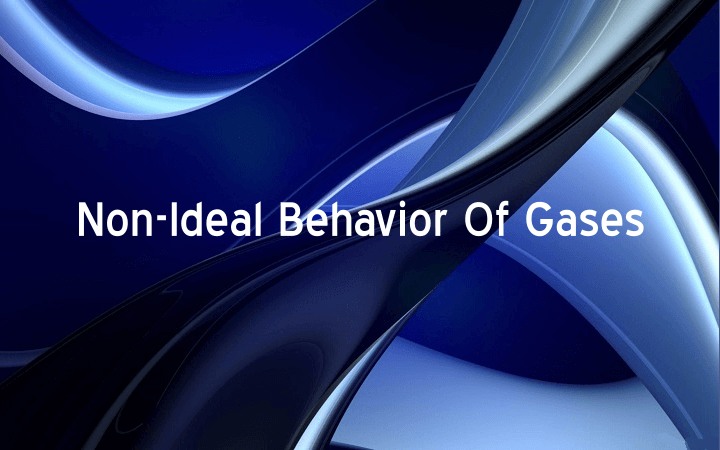## Van der Waals’ Equation for Real Gases

The general gas equation is not applicable to real gases at the all the temperature .and pressure. In order to make it applicable to real gases Van der Waals’ made correction in volume and pressure of the gases and derived an equation known as Van […]## Non-Ideal Behavior Of Gases

Ideal Gases These are the gases, which obey gas laws at all temperatures and pressures. No such gas is known. Non-Ideal gases (Real gases) These are the gases, which obey gas laws but only at high temperatures and low pressures. These gases do not obey […]## Ideal Gas Law | Combined Gas Law

Definition: The relationship, which shows the effect of simultaneous changes in pressure and temperature on the volume of given mass of a gas is called ideal gas law or combined gas law. Mathematical Form: (General gas equation) Mathematically, it is expressed as PV = nRT […]## Absolute Zero

Following statement of Charles’ law helps to derive absolute zero of temperature. “At constant pressure the volume of a given mass of an ideal gas increases or decreases by 1/273 of its original volume at 0°C for every 1°C rise or fall in temperature respectively.” […]## Charles’ Law

Introduction: In 1787, Charles found a relationship between volume and temperature of gases. He announced this relationship in the form of a law known as Charles’ law: Definition: “Volume of a given mass of a gas is directly proportional to the absolute temperature at constant […]## Boyle’s Law

Introduction: In 1662, Boyle found a relationship between volume and pressure of gases. He announced this relationship in the form of a law known as Boyle’s law. Statement: “At constant temperature, volume of a given mass of a gas is inversely proportional to pressure exerted […]